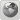Runiter Company
Would you like to react to this message? Create an account in a few clicks or log in to continue.

# Plotting the equation of tangent line to a curve##Plotting the equation of tangent line to a curve

Hi,

I want to plot the following equation of tangent line to the curve.,
My attempt:I put the following graph controls: a=5  α=v=-pi to pi, θ= u= -pi to pi, x-axis=-5 to 5, y-axis=-5 to 5, z-axis=-32 to 32.
Using the above graphing controls, I got the following graph in your Runiter graphing calculator.Is this graph correctly plotted?  If not, how to plot it correctly?

If any member of this forum  knows the correct answer to this question may reply with correct answer.Dhamnekar Winod

Posts : 3
Join date : 2020-06-03
Location : Mumbai, Maharashtra state, India.

##Re: Plotting the equation of tangent line to a curve

The original curve is a parametric equation that can be plotted simply as this:But the equation to tangent line is not something I've seen before.
If you can provide a link or description of how vector r=x/something=y/something=z/something is usually plotted then I might be able to help.

_________________
Saeid Nourian, Ph.D.
Founder of Runiter Company
http://www.runiter.comruniter

Posts : 135
Join date : 2007-12-05
Age : 39
Location : Boston, MA, USAPermissions in this forum:
You cannot reply to topics in this forum